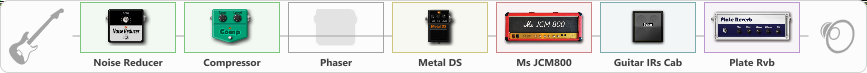Discussion in 'ToneLib-GFX presets' started by AmegoO, May 26, 2021.

Effects chain:Effect: "Noise Reducer" (Динамика / Фильтр), active - "yes"
{
"Sens" = 81
"Mode" = Hard
}

Effect: "Compressor" (Динамика / Фильтр), active - "yes"
{
"Sense" = 34
"Level" = 100
}

Effect: "Phaser" (Модуляция / Sfx), active - "no"
{
"Model" = Boss PH-3
"Speed" = 5.0
"Depth" = 100
"Reso" = 26
"Freq" = 5.0
}

Effect: "Metal DS" (Перегрузка / Искажение), active - "yes"
{
"Dist" = 80
"Bass" = 50
"Middle" = 50
"Treble" = 47
"Level" = 68
}

Effect: "Ms JCM800" (Усилители симуляторы), active - "yes"
{
"Gain" = 76
"Bass" = 67
"Middle" = 50
"Treble" = 50
"Presence" = 68
"Master" = 59
"Level (dB)" = 0
}

Effect: "Guitar IRs Cab" (Кабинеты), active - "yes"
{
"Model" = Marshall 1960A (4x12")
"Mic Position" = Middle
"Mic Distance" = Near
"Low Cut (Hz)" = 60
"Hi Cut (kHz)" = 20.0
"Mix" = 100
"Level (dB)" = 0
}

Effect: "Plate Rvb" (Реверберация), active - "yes"
{
"Time" = 4.3
"PreDelay" = 30
"LoDamp" = 17
"HiDamp" = 25
"Mix" = 42
}

Note: You will need to download and install the ToneLib-GFX software to use the preset.